SSC BOARD PAPERS IMPORTANT TOPICS COVERED FOR BOARD EXAM 2024

### Practice Set 6.3 Statistics Class 10th Mathematics Part 1 MHB Solution

Practice Set 6.3
1. The following table shows the information regarding the milk collected from farmers on…
2. Electricity used by some families is shown in the following table. Find the mode for…
3. Grouped frequency distribution of supply of milk to hotels and the number of hotels is…
4. The following frequency distribution table gives the ages of 200 patients treated in a…

###### Practice Set 6.3
Question 1.

The following table shows the information regarding the milk collected from farmers on a milk collection centre and the content of fat in the milk, measured by a lactometer. Find the mode of fat content.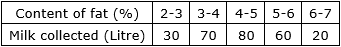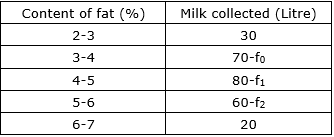⇒ Maximum amount of milk collected in class 4-5

⇒ 4-5 is modal class

L = lower limit of modal class = 4

h = class interval of modal class = 1

f1 = frequency of modal class = 80

f2 = frequency of class succeeding modal class = 60

f0 = frequency of class preceding modal class = 70

⇒ Mode =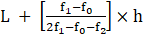⇒ Mode =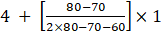⇒ Mode =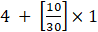⇒ Mode = 4.33 litre

Question 2.

Electricity used by some families is shown in the following table. Find the mode for use of electricity.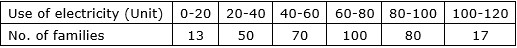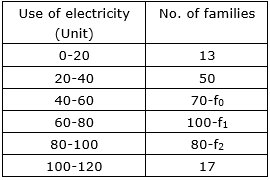⇒ Maximum amount of Electricity in class 60-80

⇒ 60-80 is modal class

L = lower limit of modal class = 60

h = class interval of modal class = 20

f1 = frequency of modal class = 100

f2 = frequency of class succeeding modal class = 80

f0 = frequency of class preceding modal class = 70

⇒ Mode =⇒ Mode =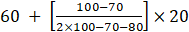⇒ Mode =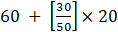⇒ Mode = 72 families

Question 3.

Grouped frequency distribution of supply of milk to hotels and the number of hotels is given in the following table. Find the mode of the supply of milk.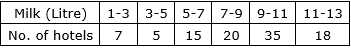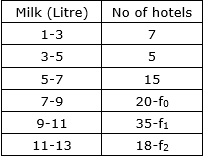⇒ Maximum amount of Electricity in class 9-11

⇒ 9-11 is modal class

L = lower limit of modal class = 9

h = class interval of modal class = 2

f1 = frequency of modal class = 35

f2 = frequency of class succeeding modal class = 18

f0 = frequency of class preceding modal class = 20

⇒ Mode =⇒ Mode =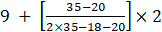⇒Mode =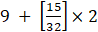⇒ Mode = 9.94 hotels

Question 4.

The following frequency distribution table gives the ages of 200 patients treated in a hospital in a week. Find the mode of ages of the patients.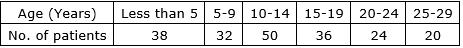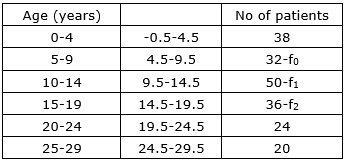⇒ Maximum amount of Electricity in class 9.5-14.5

⇒ 9.5-14.5 is modal class

L = lower limit of modal class = 9.5

h = class interval of modal class = 5

f1 = frequency of modal class = 50

f2 = frequency of class succeeding modal class = 36

f0 = frequency of class preceding modal class = 32

⇒ Mode =⇒ Mode =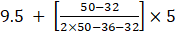⇒ Mode =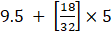⇒ Mode = 12.31 years

Mode age of the patient 12.31 years